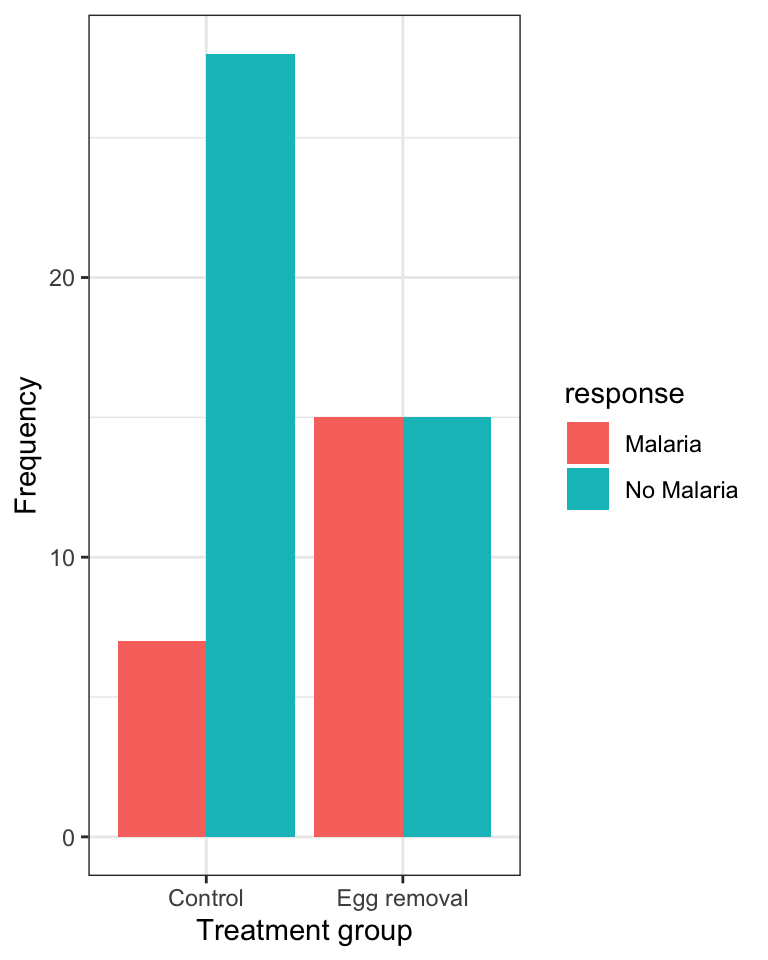## 8.3 Visualizing association between two numeric variables

We use a scatterplot to show association between two numerical variables.

We’ll use the ggplot function that we’ve seen before, along with geom_point to construct a scatterplot.

We’ll provide an example using the penguins dataset, examining how bill depth and length are associated among the penguins belonging to the Adelie species.

As shown in the tutorial on preparing and formatting assignments, we can use the filter function from the dplyr package to easily subset datasets according to some criterion, such as belonging to a specific category.

penguins %>%
ggplot(aes(x = bill_length_mm, y = bill_depth_mm)) +
geom_point(shape = 1) +
xlab("Bill length (mm)") +
ylab("Bill depth (mm)") +
theme_bw()Figure 8.5: Scatterplot of the association between bill length and depth among 151 Adelie penguins

In the code chunk above, we have:

• the input tibble penguins followed by the pipe (“%>%”)
• the filter function with the criterion used for subsetting, specifically any cases in which the “species” categorical variable equals “Adelie”
• then we provide the ggplot function and its aes argument, specifying the x- and y- variables to be used
• then we use geom_point to tell R to create a scatterplot using points, and specifically “shape = 1” denotes hollow circles
• then we have x and y labels, followed by the theme_bw function telling R to use black and white theme

Notice that the figure caption indicates the number of observations (sample size) used in the plot. In a previous tutorial it was emphasized that one needs to be careful in tallying the actual number of observations being used in a graph or when calculating descriptive statistics. For example, there is one missing value (“NA”) in the bill measurements for the Adelie penguins, hence the sample size of 151 instead of 152.

Recall that you can use the skim or skim_without_charts functions to get an overview of a dataset or of a single variable in a dataset, and to figure out how many missing values there are for each variable. You can also use the summarise function, as described previously.

### 8.3.1 Interpreting and describing a scatterplot

Things to report when describing a scatterplot:

• is there an association? A “shotgun blast” pattern indicates no. If there is an association, is it positive or negative?
• if there is an association, is it weak, moderate, or strong?
• is the association linear? If not, is there a different pattern like concave down?
• are there any outlier observations that lie far from the general trend?

In the scatterplot above, bill length and depth are positively associated, and the association is moderately strong. There are no observations that are strongly inconsistent with the general trend, though one individual with bill length of around 35mm and depth of around 21mm may be somewhat unusual.

1. Using the penguins dataset, create a scatterplot of flipper length in relation to body mass, and provide an appropriate figure caption.